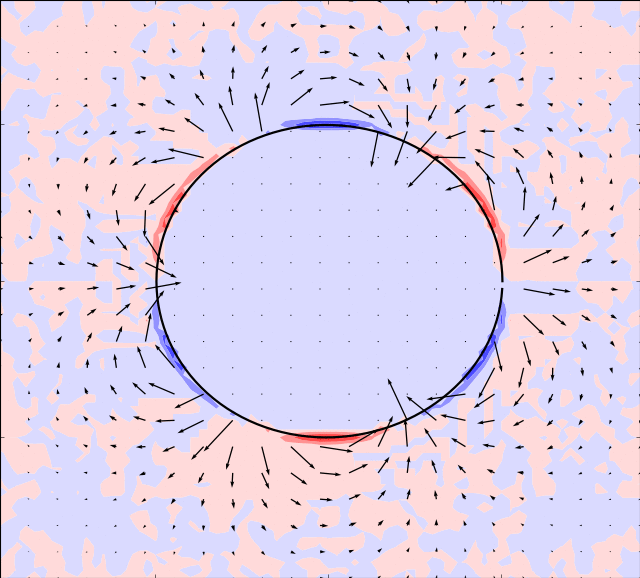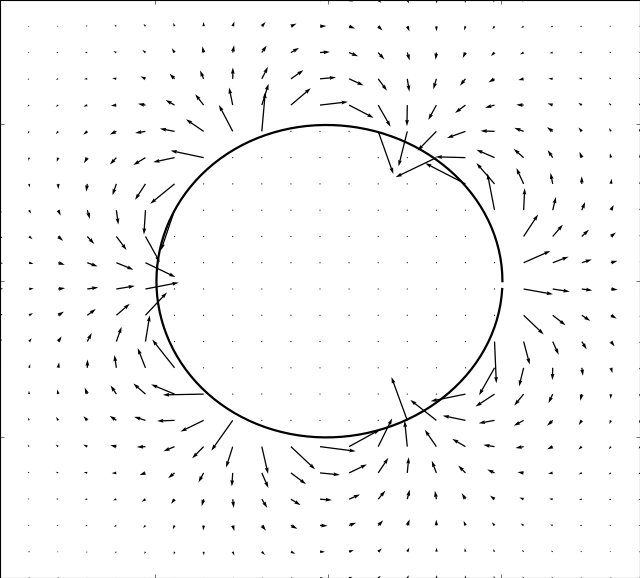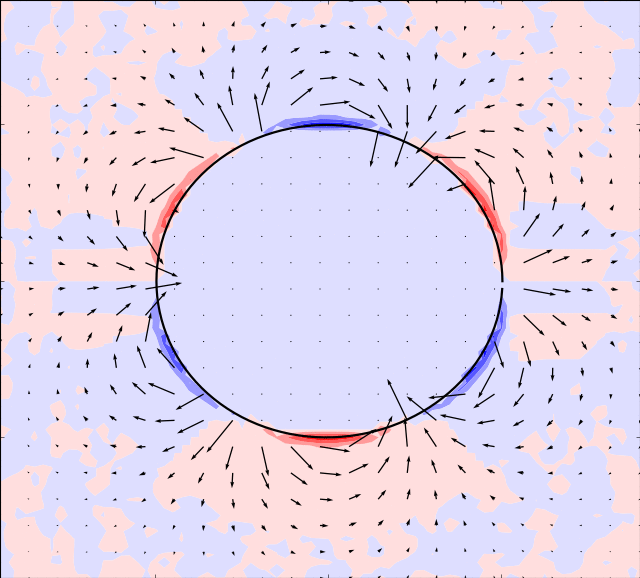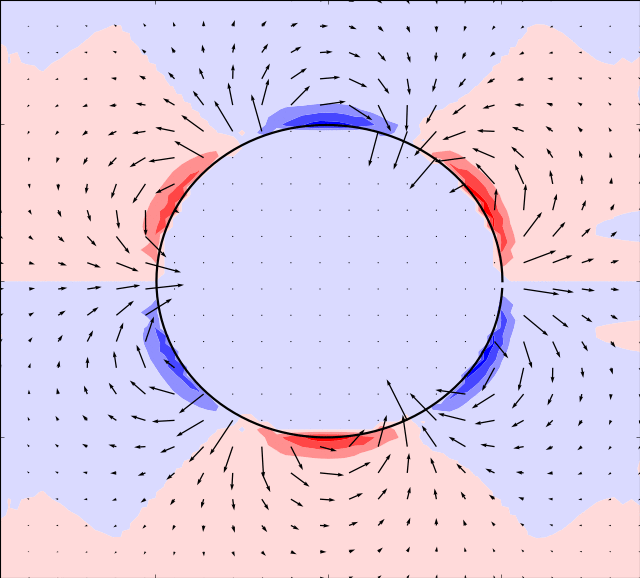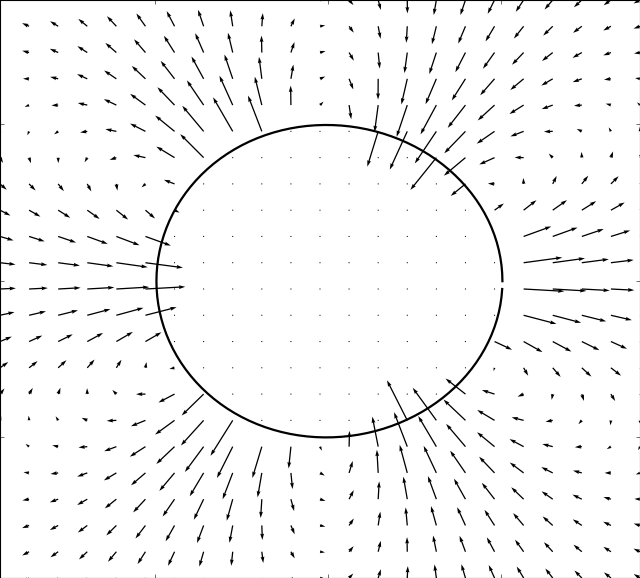# Mason & Burdick Amoeba¶

Here we take the example given by [MB99]. The boundary is simulated by:By using an appropriate time evolution of the two shape modes we can achieve forward motion.In the simulations below, the parameter is set toand the following evolution in time of the shape modes is usedIn order for the immersed boundary method to converge we must also ensure that the area enclosed by the boundary remains constant. This can be achieved by adding a normalization:whereCode

```shapevariables = (
lambda t: 1-cos(t),
lambda t: sin(t),
)

epsilon = 0.1
R = lambda s,q: 1 + epsilon*(q*cos(2*s) + q*cos(3*s))
N = lambda s,q: sqrt(1+0.5*epsilon**2*(q**2+q**2))
X = lambda s,q: R(s,q)*cos(s)/N(s,q)
Y = lambda s,q: R(s,q)*sin(s)/N(s,q)
bodies = (
(X,Y),
)
```